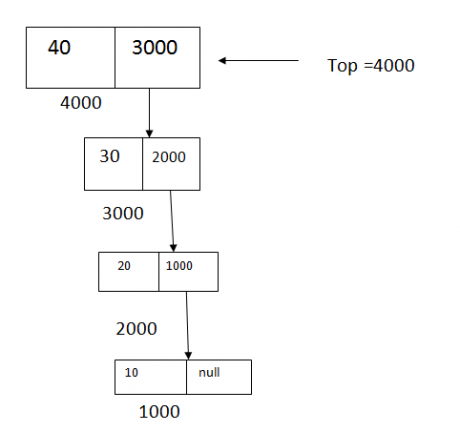Stack using Linked List in Data Structures
Ratings:
(4)
Views:0Another way to represent a stack is by using a linked list. A stack can be represented by using nodes of the linked list. Each node contains two fields: data(info) and next(link) The data field of each node contains an item in the stack and the corresponding next field points to the node containing the next item in the stack The top refers to the topmost node (The last item inserted) in the stack. The empty stack is represented by setting top to nut. Because of the way the nodes are pointing, push and pop operations are easy to accomplish.## Program to implement stack using linked list

``````# include <stdio.h>
# include <conio.h>
# include <stdlib.h>
struct Node
{
int data;
struct node *top=NULL;
void insertion(int x)
{
struct node *temp;
temp=(struct node *) malloc (size of  (structure node));
temp 7 data =x;
top=temp;
}
int deletion ()
{
struct node  *temp;
int x;
if(cop==NULL)
return -1;
else
{
temp=top;
x=temp 7 data;
free(temp);
return x;
}
void display()
{
struct node *temp;
if(top=NULL)
printf(“stack is empty n”);
else
{
printf(“The contents are “ n”);
temp=top;
while(temp!=NULL)
{
printf(“%5d”,temp7data);
}
}
}
void main()
{
int ch, x;
clrscr();
printf(“1.INSERTION n”);
printf(“2. DELETION n”);
printf(“3. DISPLAY  The stack n”);
printf(“ 4. EXIT n”);
do
{
printf(“Enter ur choice : n”);
scanf(“%d”, &ch);
switch(ch)
{
case 1 :
printf(“Enter Data elements : n”)       ;
scanf(“%d”,&x);
insertion(x);
case 2 :
x=deletion();
if(x==-1)
printf(“STACK is EMPTY n”)                 ;
else
printf(“ The Deleted value is : %d n”,x);
break;
case 3:
display ();
break;
case 4:
Exit(0);
}
} while(ch<=4);
getch();
}``````